Скачать презентацию Introduction to Survival Analysis Kristin Sainani Ph D

f1aa517a8f28a9e03a77e14df9b3402e.ppt

• Количество слайдов: 70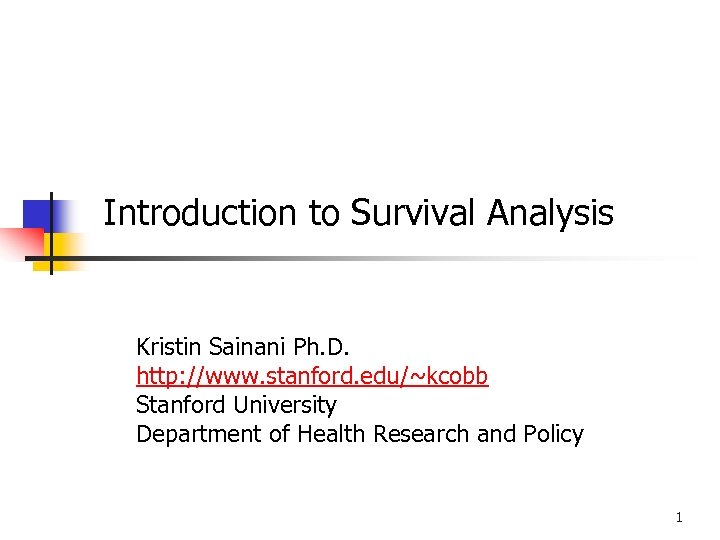Introduction to Survival Analysis Kristin Sainani Ph. D. http: //www. stanford. edu/~kcobb Stanford University Department of Health Research and Policy 1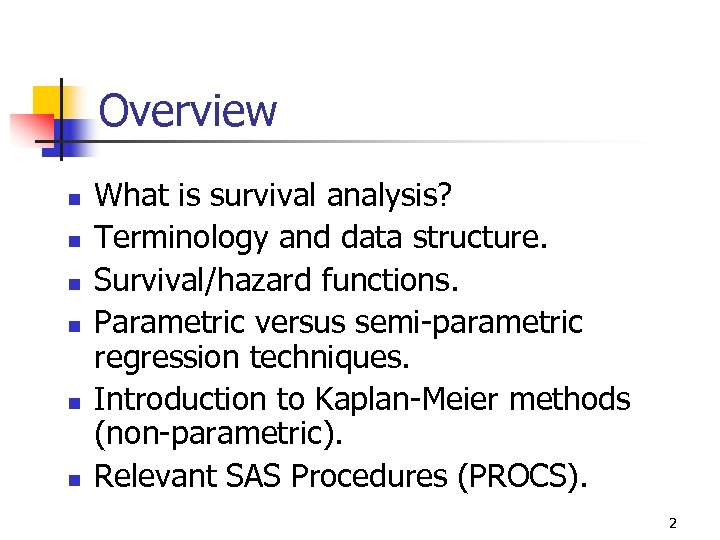Overview n n n What is survival analysis? Terminology and data structure. Survival/hazard functions. Parametric versus semi-parametric regression techniques. Introduction to Kaplan-Meier methods (non-parametric). Relevant SAS Procedures (PROCS). 2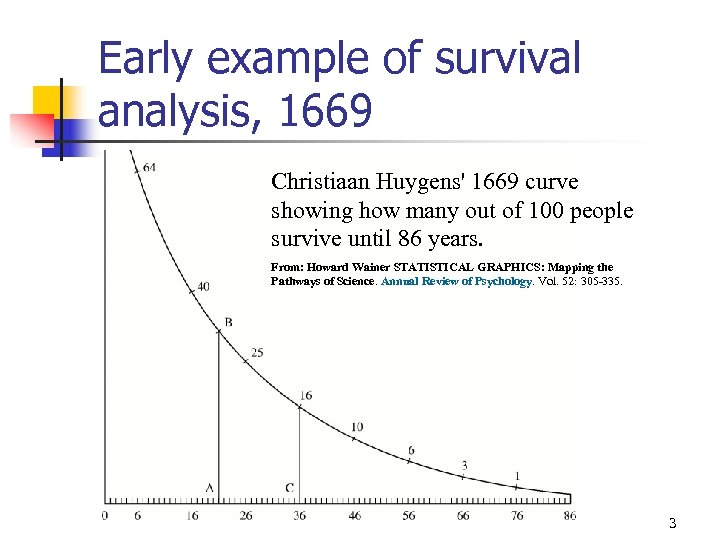Early example of survival analysis, 1669 Christiaan Huygens' 1669 curve showing how many out of 100 people survive until 86 years. From: Howard Wainer STATISTICAL GRAPHICS: Mapping the Pathways of Science. Annual Review of Psychology. Vol. 52: 305 -335. 3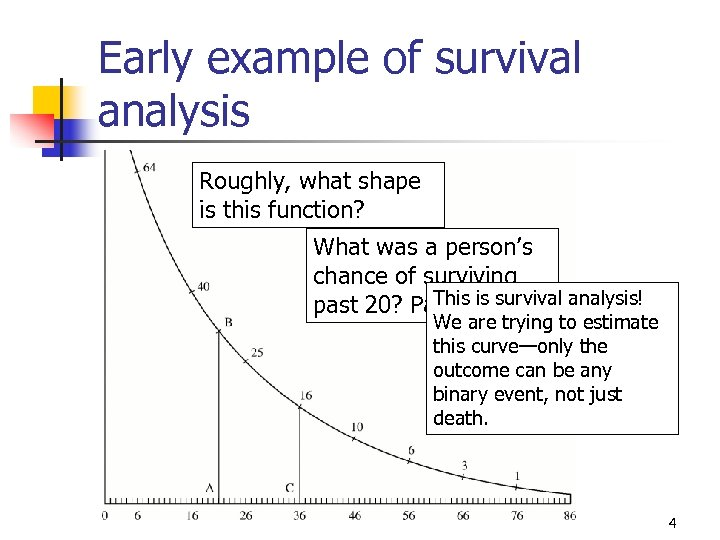Early example of survival analysis Roughly, what shape is this function? What was a person’s chance of surviving This is survival analysis! past 20? Past 36? We are trying to estimate this curve—only the outcome can be any binary event, not just death. 4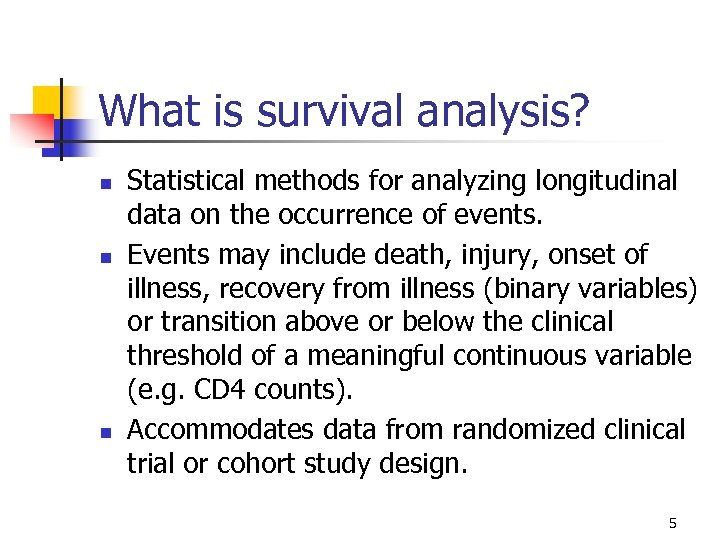What is survival analysis? n n n Statistical methods for analyzing longitudinal data on the occurrence of events. Events may include death, injury, onset of illness, recovery from illness (binary variables) or transition above or below the clinical threshold of a meaningful continuous variable (e. g. CD 4 counts). Accommodates data from randomized clinical trial or cohort study design. 5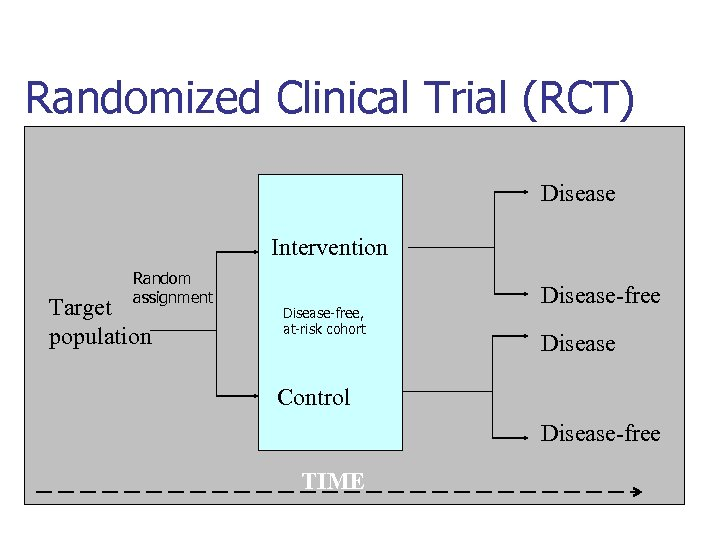Randomized Clinical Trial (RCT) Disease Intervention Random assignment Target population Disease-free, at-risk cohort Disease-free Disease Control Disease-free TIME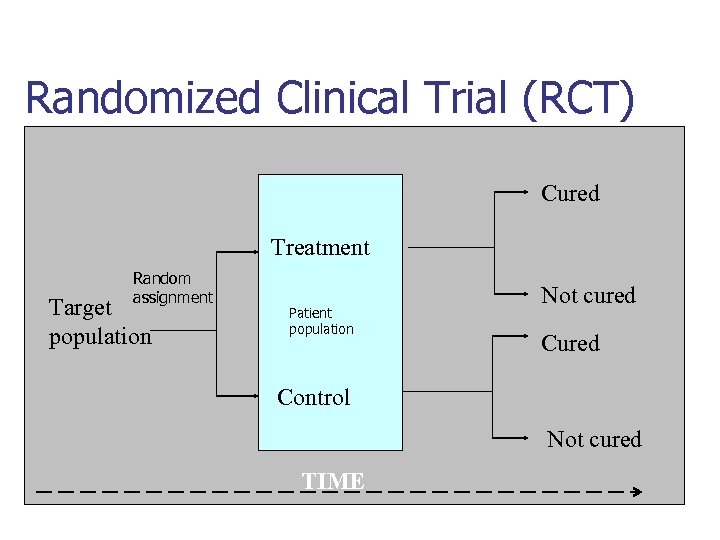Randomized Clinical Trial (RCT) Cured Treatment Random assignment Target population Patient population Not cured Control Not cured TIME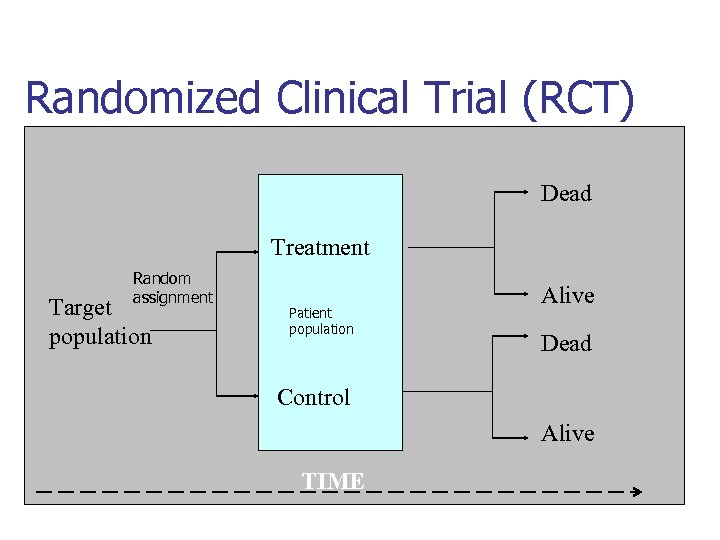Randomized Clinical Trial (RCT) Dead Treatment Random assignment Target population Patient population Alive Dead Control Alive TIME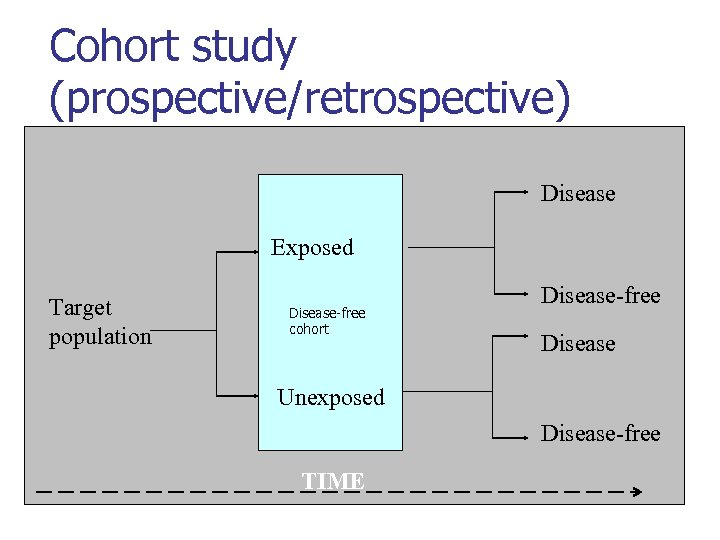Cohort study (prospective/retrospective) Disease Exposed Target population Disease-free cohort Disease-free Disease Unexposed Disease-free TIME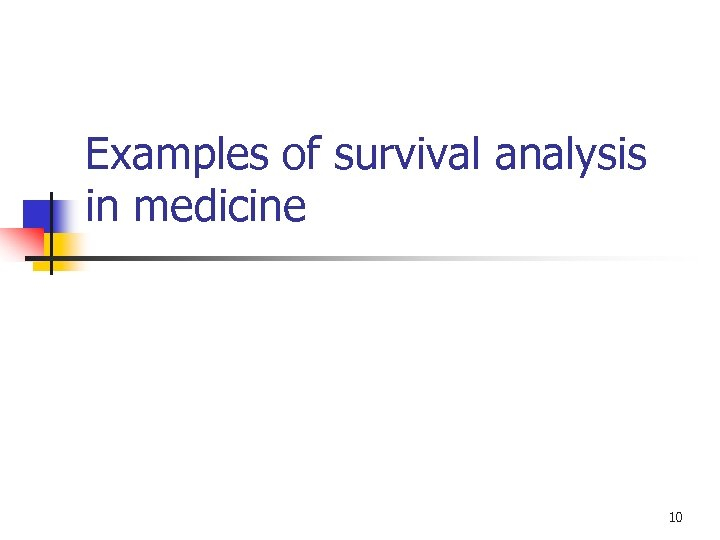Examples of survival analysis in medicine 10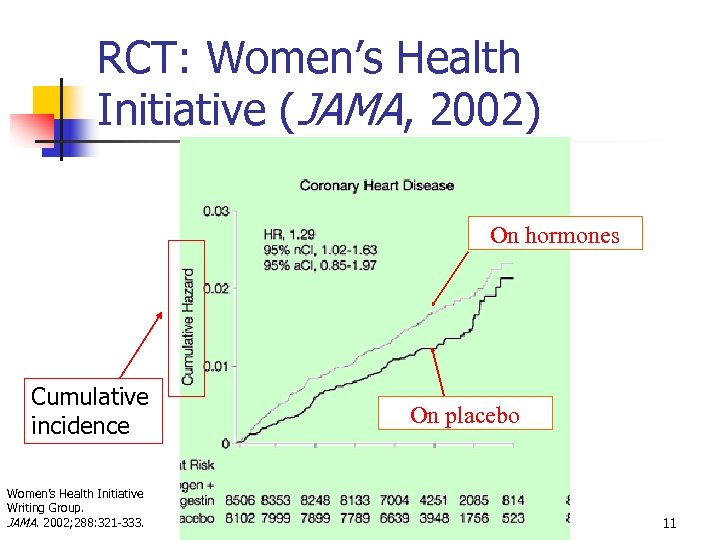RCT: Women’s Health Initiative (JAMA, 2002) On hormones Cumulative incidence Women’s Health Initiative Writing Group. JAMA. 2002; 288: 321 -333. On placebo 11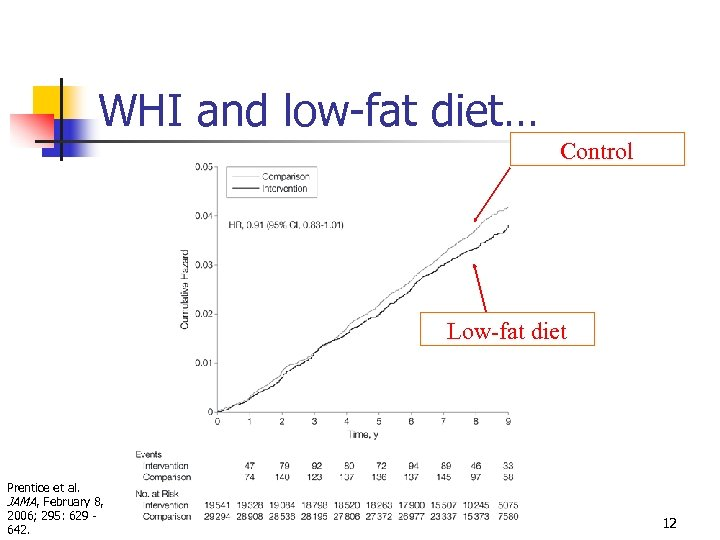WHI and low-fat diet… Control Low-fat diet Prentice et al. JAMA, February 8, 2006; 295: 629 - 642. 12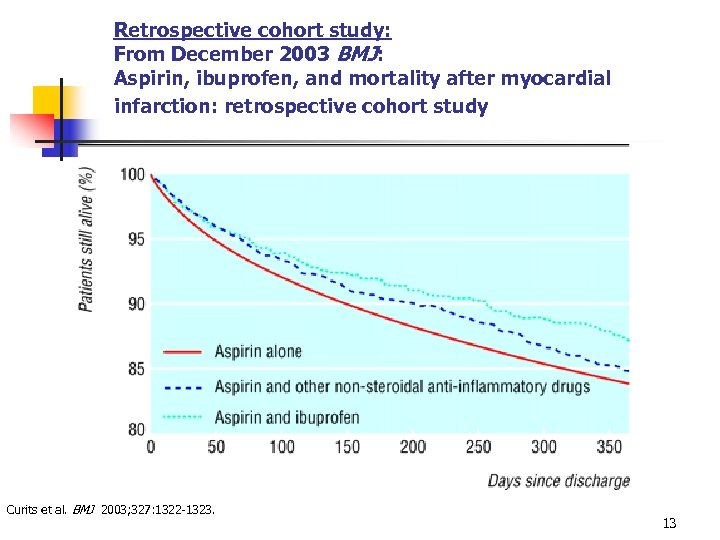Retrospective cohort study: From December 2003 BMJ: Aspirin, ibuprofen, and mortality after myocardial infarction: retrospective cohort study Curits et al. BMJ 2003; 327: 1322 -1323. 13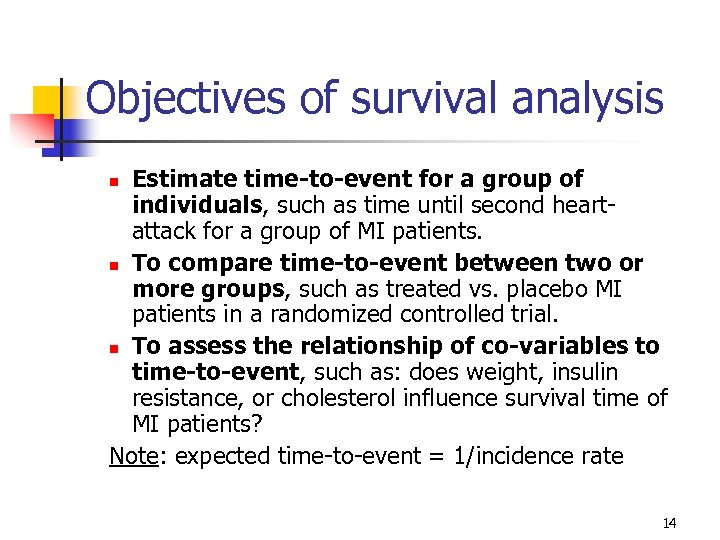Objectives of survival analysis Estimate time-to-event for a group of individuals, such as time until second heartattack for a group of MI patients. n To compare time-to-event between two or more groups, such as treated vs. placebo MI patients in a randomized controlled trial. n To assess the relationship of co-variables to time-to-event, such as: does weight, insulin resistance, or cholesterol influence survival time of MI patients? Note: expected time-to-event = 1/incidence rate n 14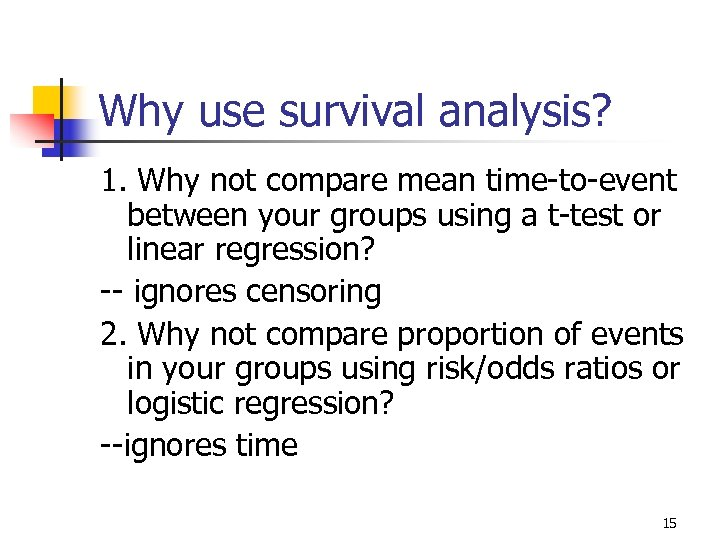Why use survival analysis? 1. Why not compare mean time-to-event between your groups using a t-test or linear regression? -- ignores censoring 2. Why not compare proportion of events in your groups using risk/odds ratios or logistic regression? --ignores time 15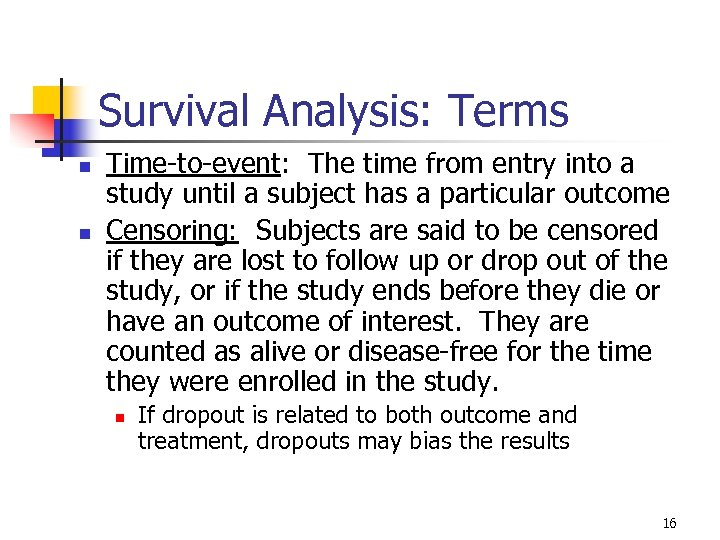Survival Analysis: Terms n n Time-to-event: The time from entry into a study until a subject has a particular outcome Censoring: Subjects are said to be censored if they are lost to follow up or drop out of the study, or if the study ends before they die or have an outcome of interest. They are counted as alive or disease-free for the time they were enrolled in the study. n If dropout is related to both outcome and treatment, dropouts may bias the results 16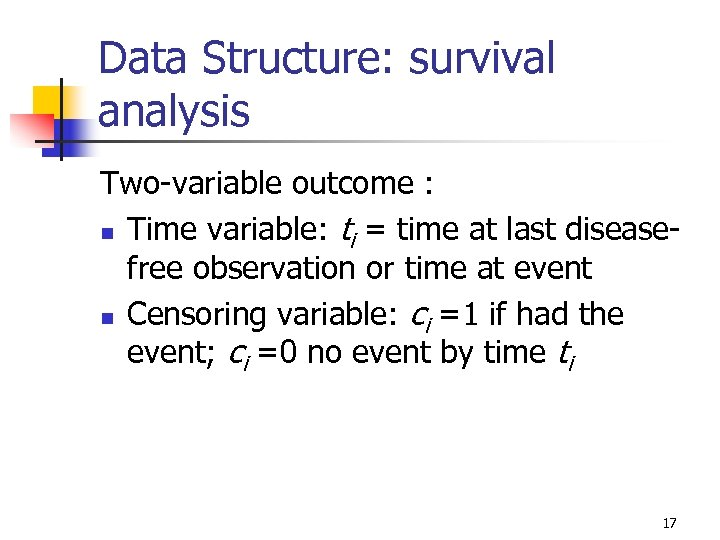Data Structure: survival analysis Two-variable outcome : n Time variable: ti = time at last diseasefree observation or time at event n Censoring variable: ci =1 if had the event; ci =0 no event by time ti 17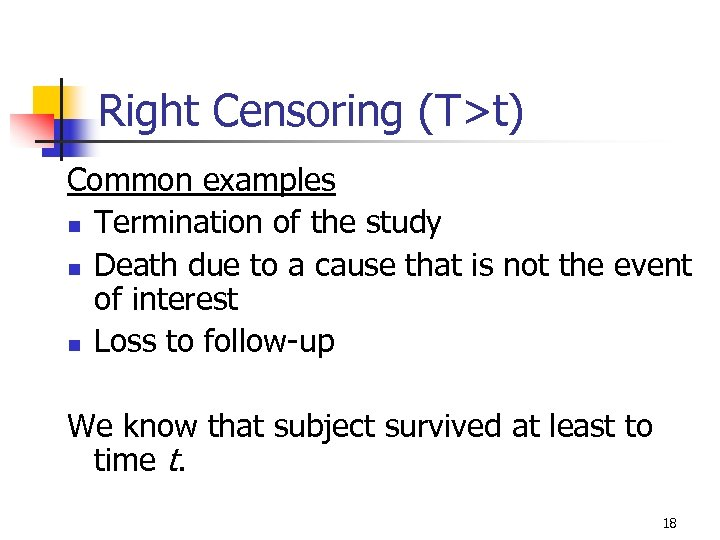Right Censoring (T>t) Common examples n Termination of the study n Death due to a cause that is not the event of interest n Loss to follow-up We know that subject survived at least to time t. 18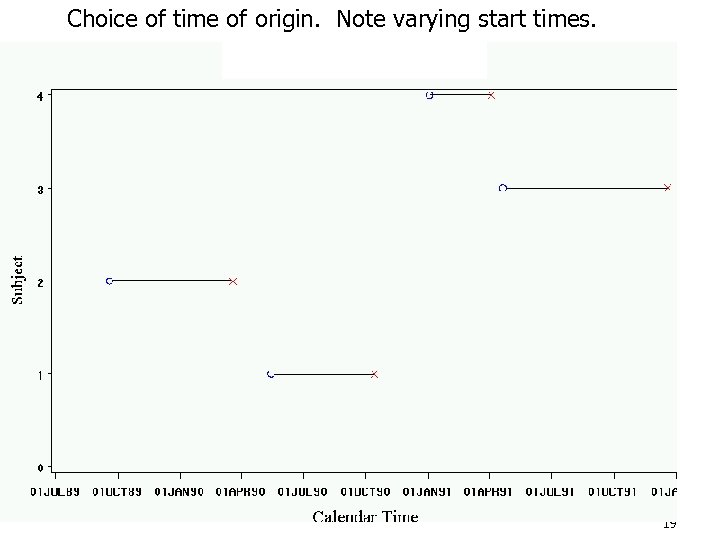Choice of time of origin. Note varying start times. 19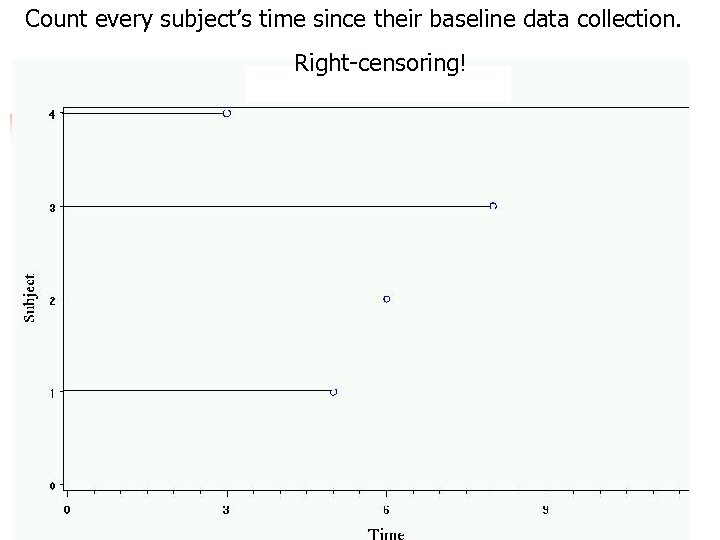Count every subject’s time since their baseline data collection. Right-censoring! 20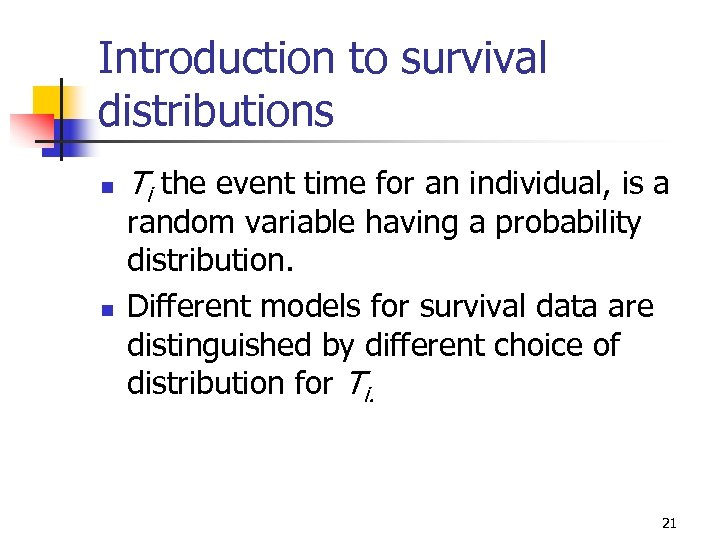Introduction to survival distributions n n Ti the event time for an individual, is a random variable having a probability distribution. Different models for survival data are distinguished by different choice of distribution for Ti. 21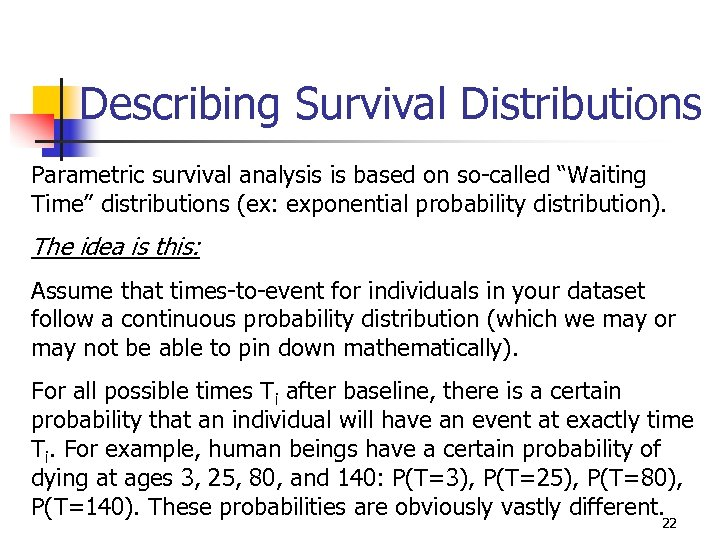Describing Survival Distributions Parametric survival analysis is based on so-called “Waiting Time” distributions (ex: exponential probability distribution). The idea is this: Assume that times-to-event for individuals in your dataset follow a continuous probability distribution (which we may or may not be able to pin down mathematically). For all possible times Ti after baseline, there is a certain probability that an individual will have an event at exactly time Ti. For example, human beings have a certain probability of dying at ages 3, 25, 80, and 140: P(T=3), P(T=25), P(T=80), P(T=140). These probabilities are obviously vastly different. 22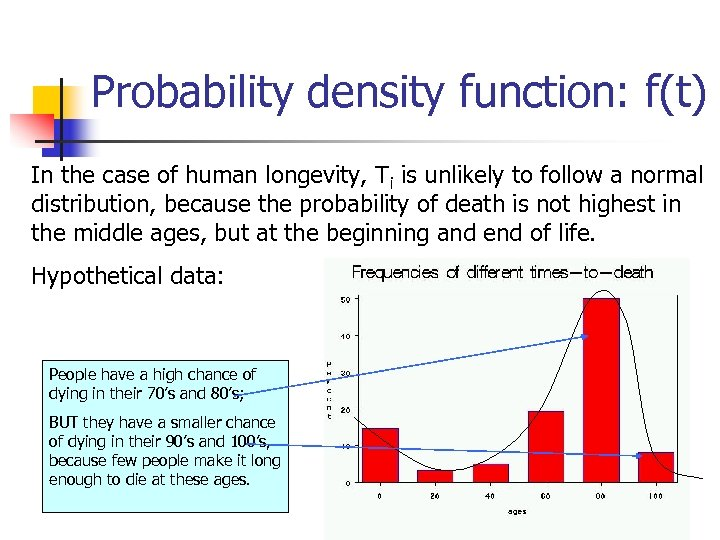Probability density function: f(t) In the case of human longevity, Ti is unlikely to follow a normal distribution, because the probability of death is not highest in the middle ages, but at the beginning and end of life. Hypothetical data: People have a high chance of dying in their 70’s and 80’s; BUT they have a smaller chance of dying in their 90’s and 100’s, because few people make it long enough to die at these ages. 23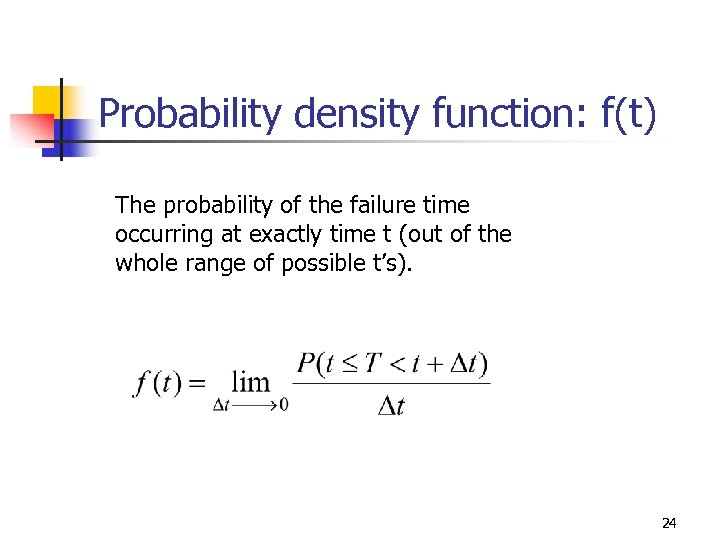Probability density function: f(t) The probability of the failure time occurring at exactly time t (out of the whole range of possible t’s). 24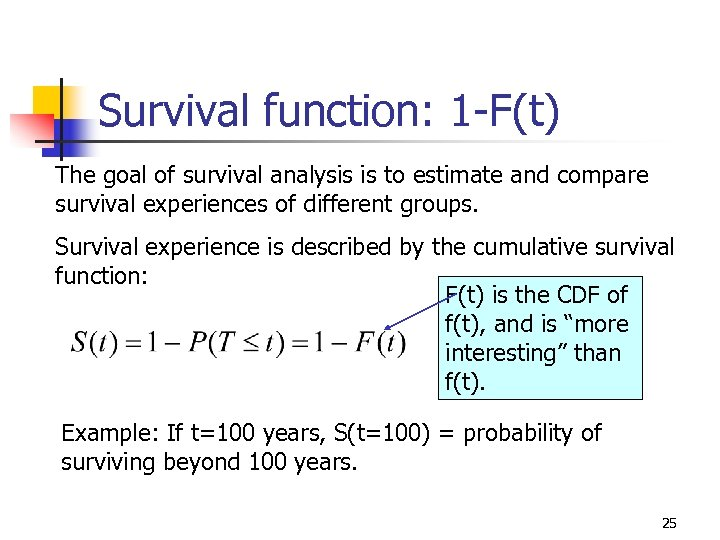Survival function: 1 -F(t) The goal of survival analysis is to estimate and compare survival experiences of different groups. Survival experience is described by the cumulative survival function: F(t) is the CDF of f(t), and is “more interesting” than f(t). Example: If t=100 years, S(t=100) = probability of surviving beyond 100 years. 25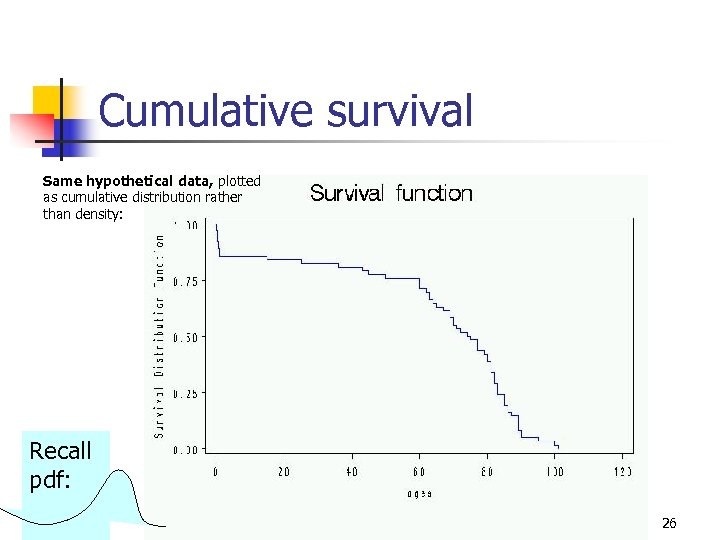Cumulative survival Same hypothetical data, plotted as cumulative distribution rather than density: Recall pdf: 26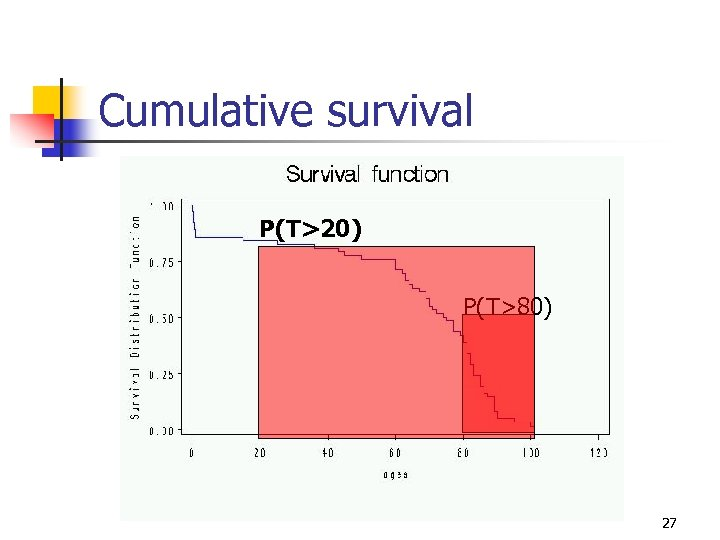Cumulative survival P(T>20) P(T>80) 27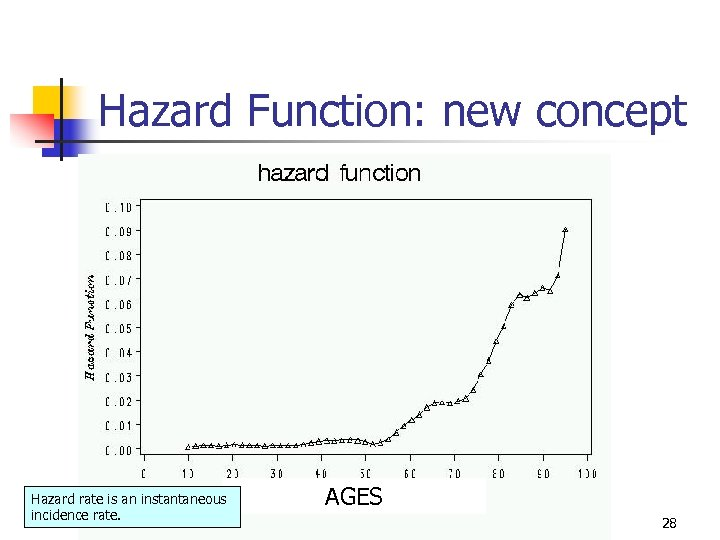Hazard Function: new concept Hazard rate is an instantaneous incidence rate. AGES 28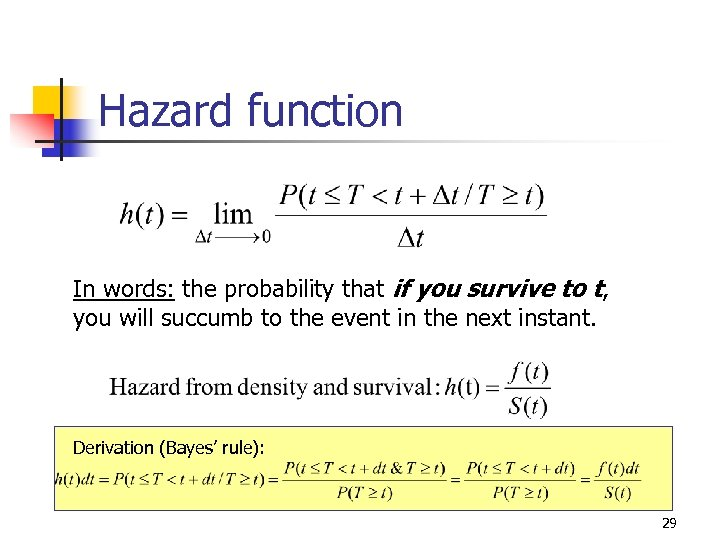Hazard function In words: the probability that if you survive to t, you will succumb to the event in the next instant. Derivation (Bayes’ rule): 29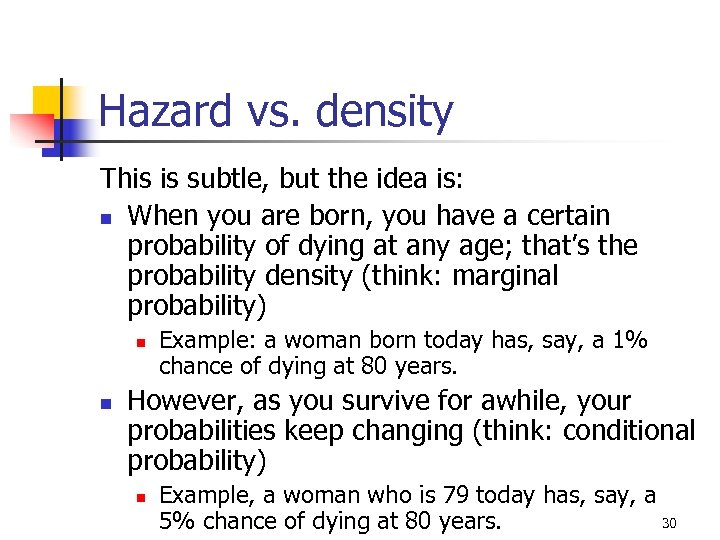Hazard vs. density This is subtle, but the idea is: n When you are born, you have a certain probability of dying at any age; that’s the probability density (think: marginal probability) n n Example: a woman born today has, say, a 1% chance of dying at 80 years. However, as you survive for awhile, your probabilities keep changing (think: conditional probability) n Example, a woman who is 79 today has, say, a 30 5% chance of dying at 80 years.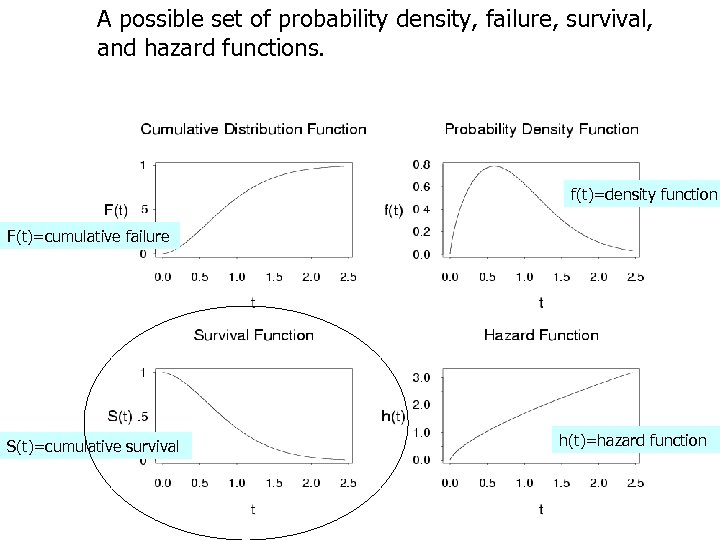A possible set of probability density, failure, survival, and hazard functions. f(t)=density function F(t)=cumulative failure S(t)=cumulative survival h(t)=hazard function 31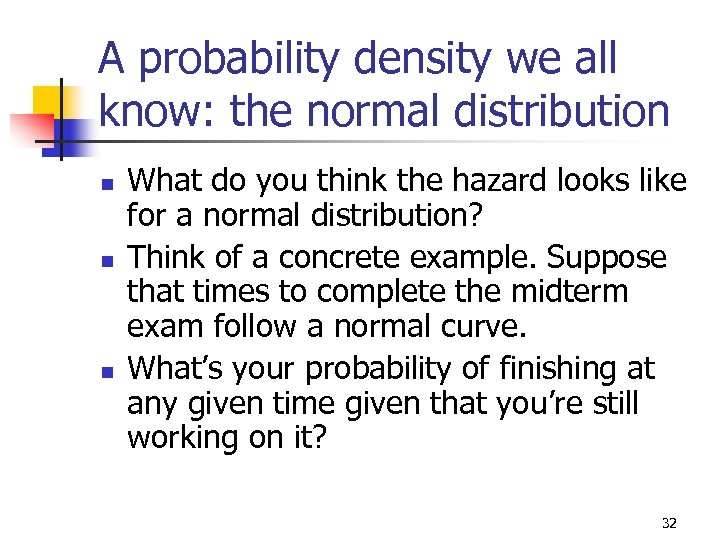A probability density we all know: the normal distribution n What do you think the hazard looks like for a normal distribution? Think of a concrete example. Suppose that times to complete the midterm exam follow a normal curve. What’s your probability of finishing at any given time given that you’re still working on it? 32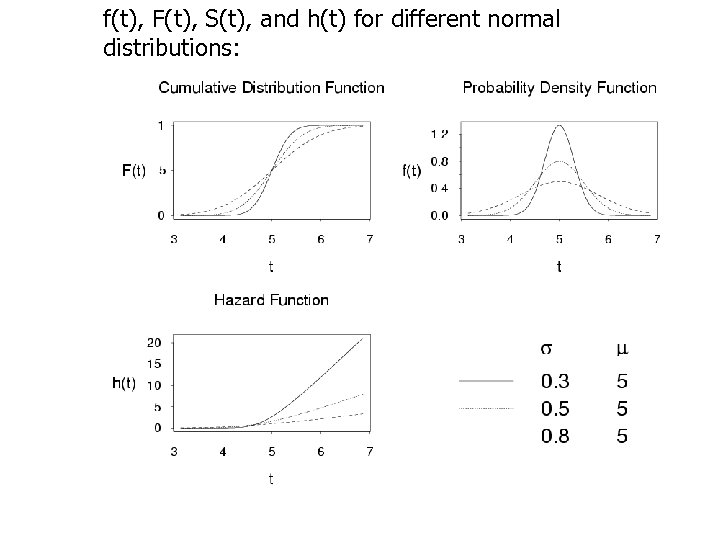f(t), F(t), S(t), and h(t) for different normal distributions: 33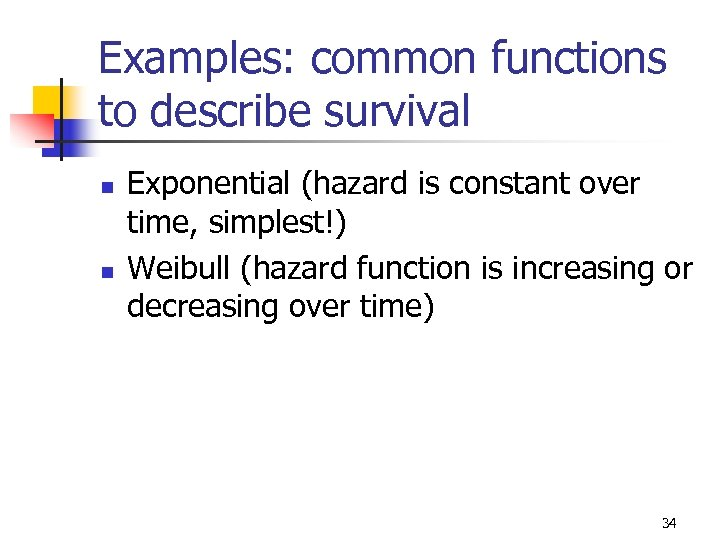Examples: common functions to describe survival n n Exponential (hazard is constant over time, simplest!) Weibull (hazard function is increasing or decreasing over time) 34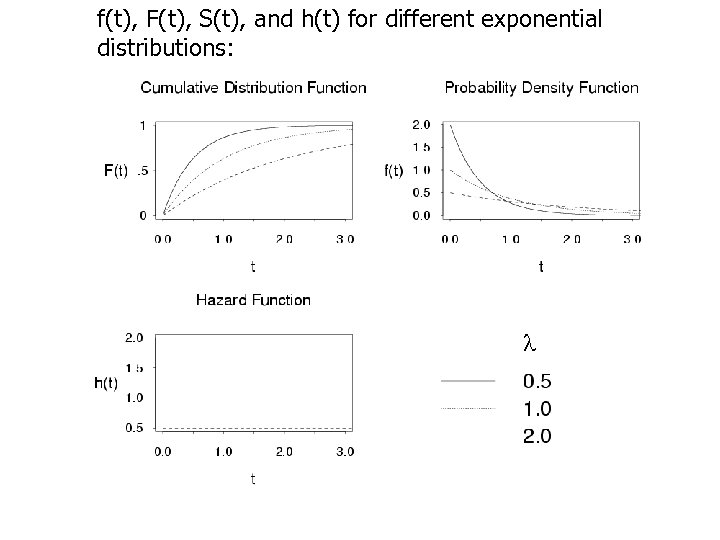f(t), F(t), S(t), and h(t) for different exponential distributions: 35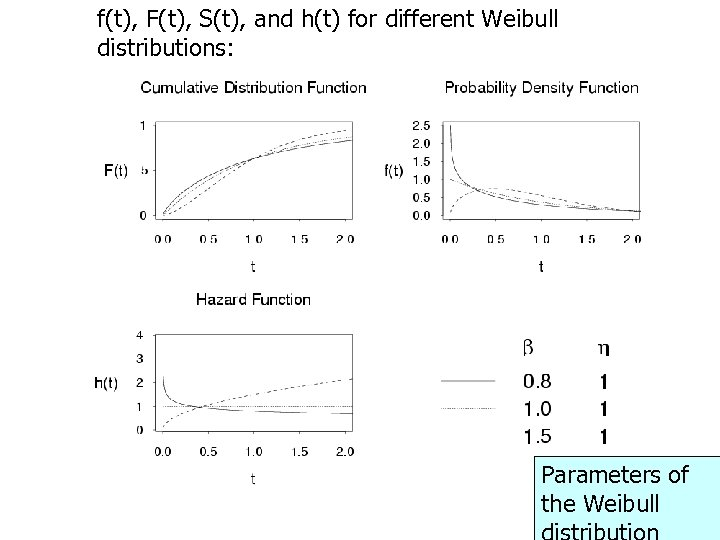f(t), F(t), S(t), and h(t) for different Weibull distributions: Parameters of the Weibull 36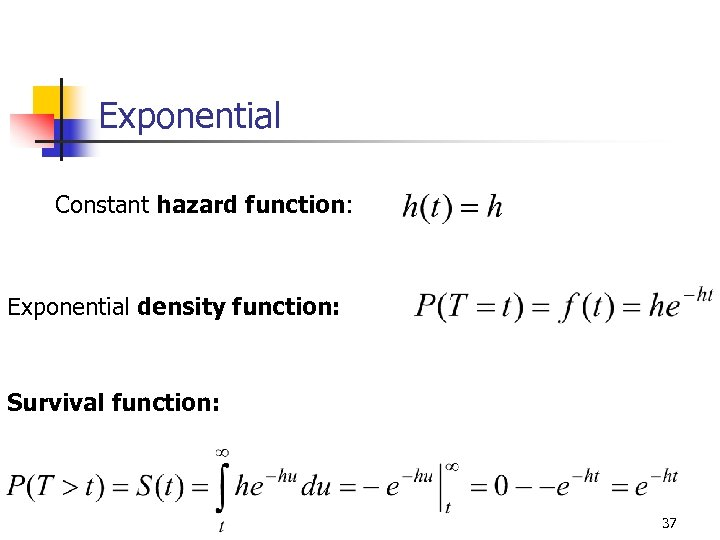Exponential Constant hazard function: Exponential density function: Survival function: 37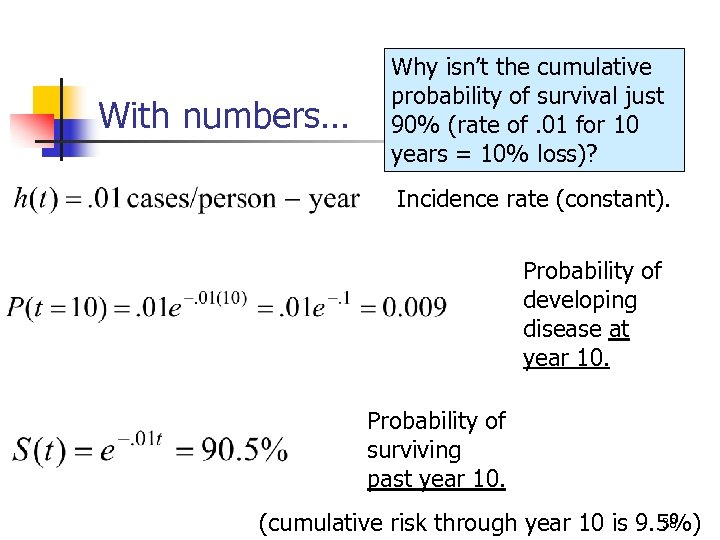With numbers… Why isn’t the cumulative probability of survival just 90% (rate of. 01 for 10 years = 10% loss)? Incidence rate (constant). Probability of developing disease at year 10. Probability of surviving past year 10. 38 (cumulative risk through year 10 is 9. 5%)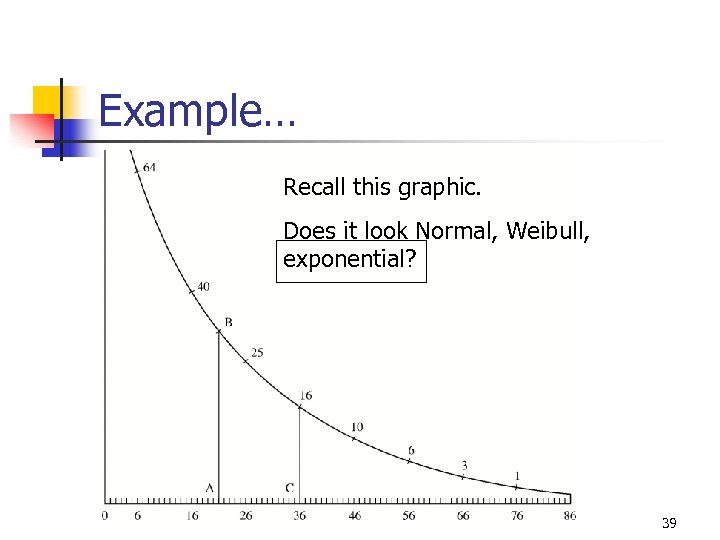Example… Recall this graphic. Does it look Normal, Weibull, exponential? 39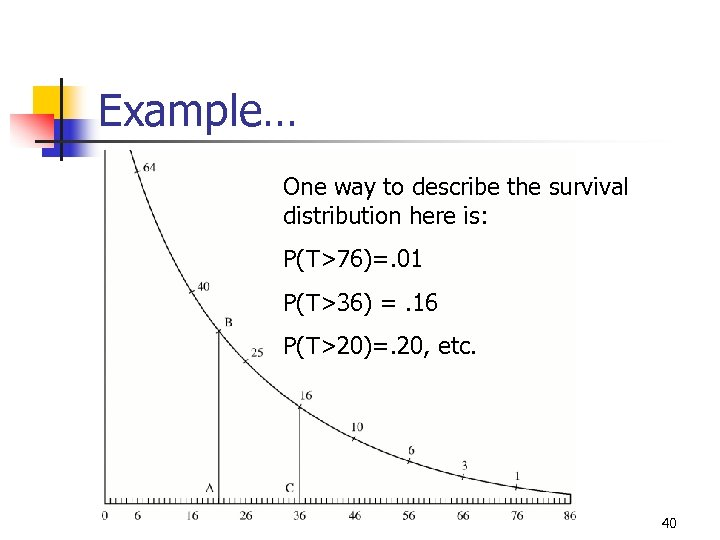Example… One way to describe the survival distribution here is: P(T>76)=. 01 P(T>36) =. 16 P(T>20)=. 20, etc. 40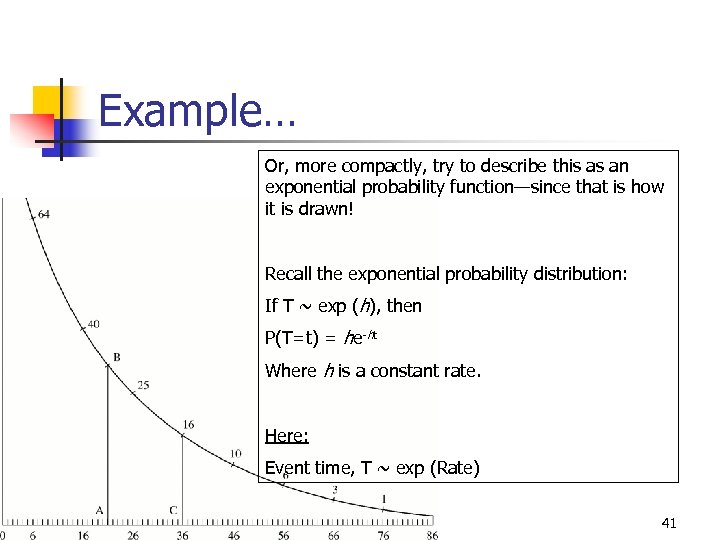Example… Or, more compactly, try to describe this as an exponential probability function—since that is how it is drawn! Recall the exponential probability distribution: If T ~ exp (h), then P(T=t) = he-ht Where h is a constant rate. Here: Event time, T ~ exp (Rate) 41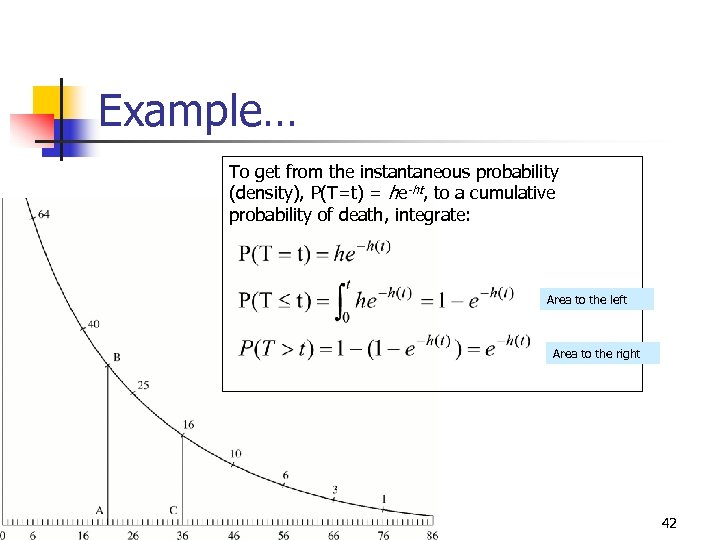Example… To get from the instantaneous probability (density), P(T=t) = he-ht, to a cumulative probability of death, integrate: Area to the left Area to the right 42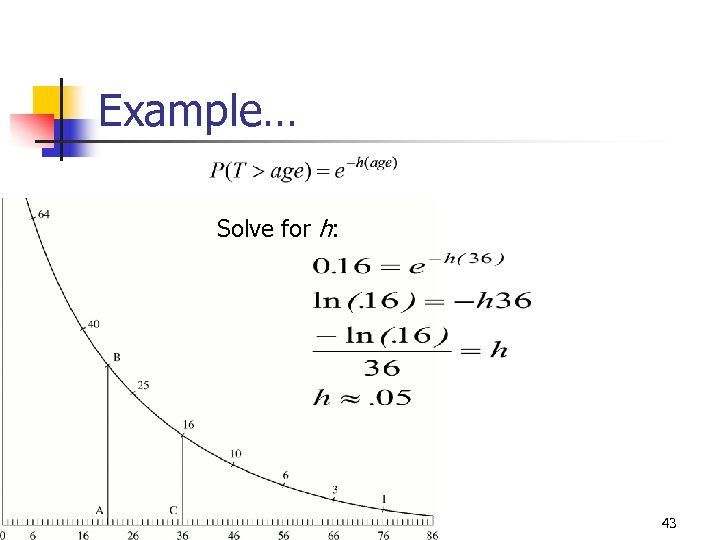Example… Solve for h: 43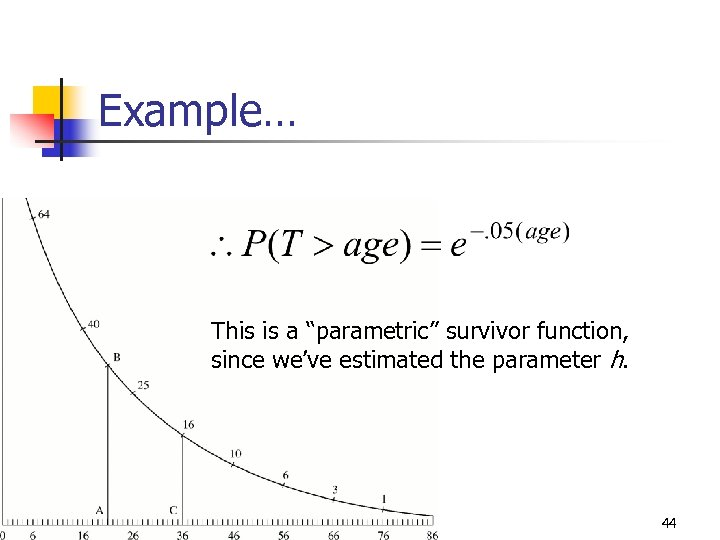Example… This is a “parametric” survivor function, since we’ve estimated the parameter h. 44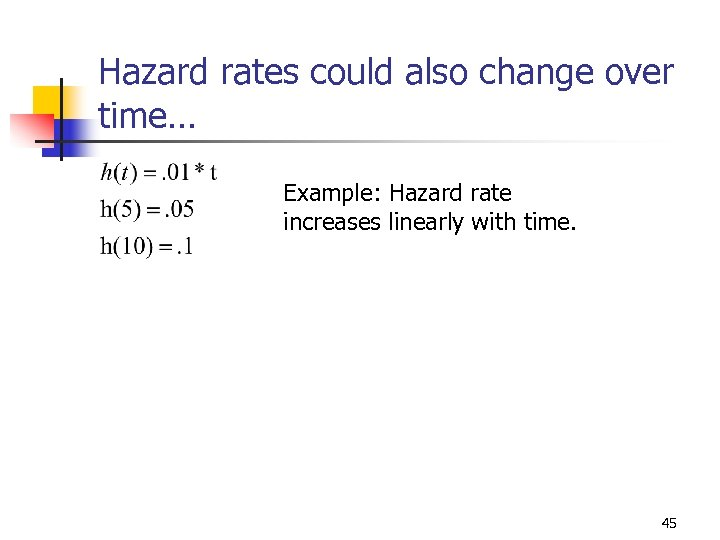Hazard rates could also change over time… Example: Hazard rate increases linearly with time. 45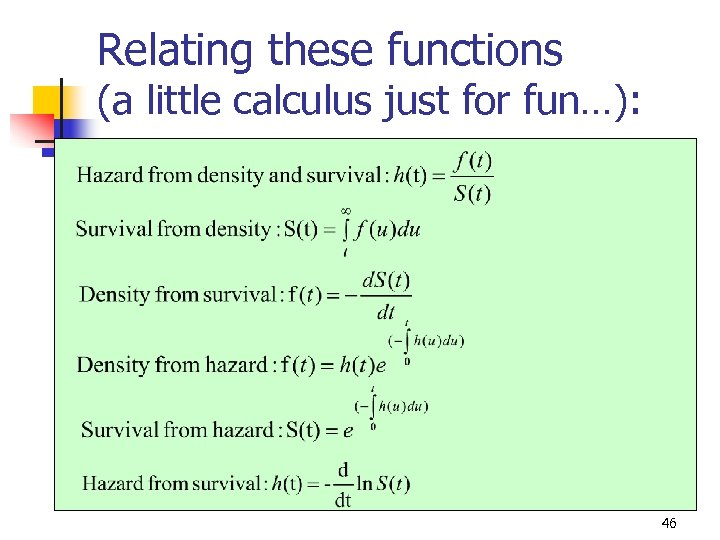Relating these functions (a little calculus just for fun…): 46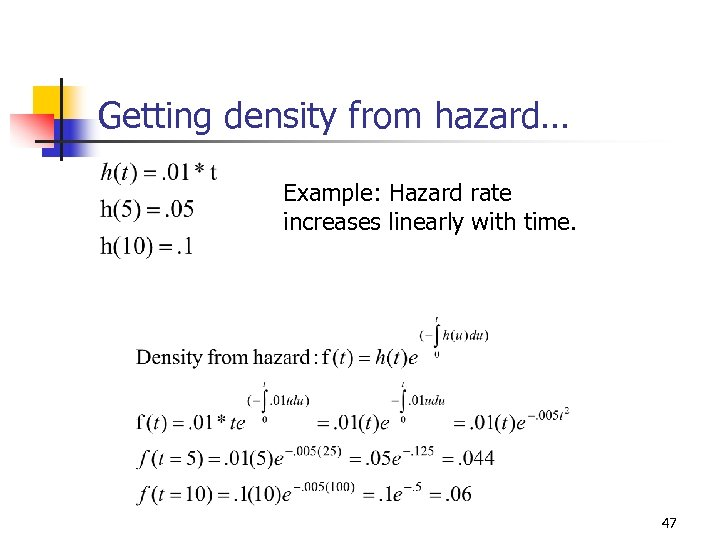Getting density from hazard… Example: Hazard rate increases linearly with time. 47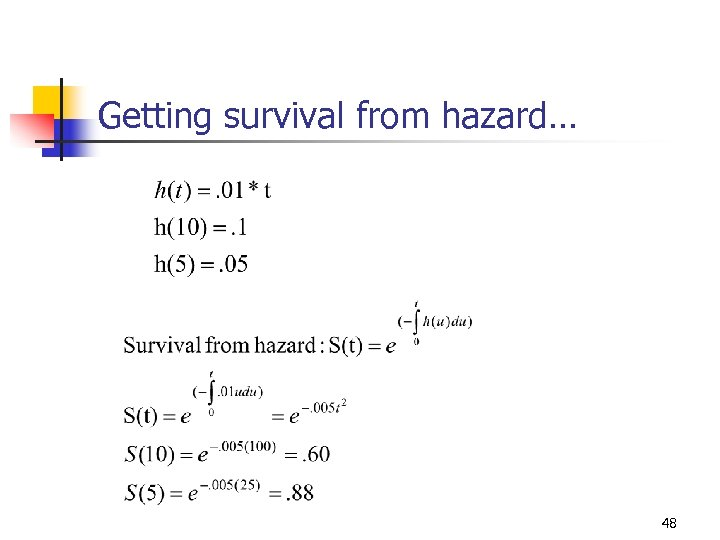Getting survival from hazard… 48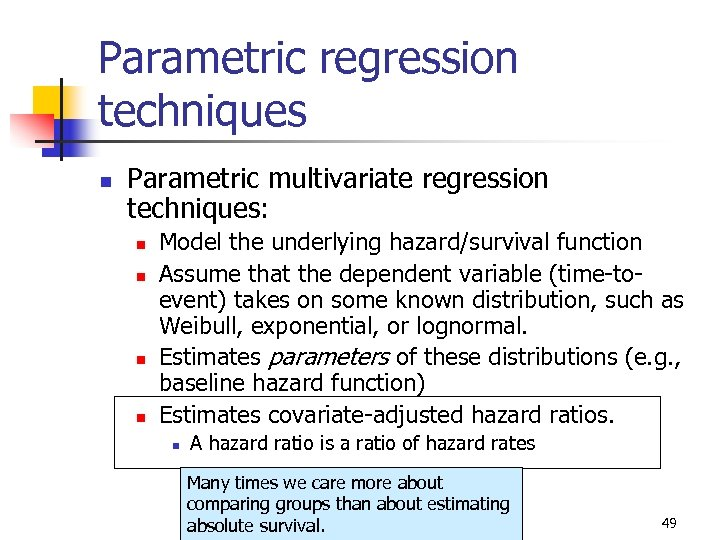Parametric regression techniques n Parametric multivariate regression techniques: n n Model the underlying hazard/survival function Assume that the dependent variable (time-toevent) takes on some known distribution, such as Weibull, exponential, or lognormal. Estimates parameters of these distributions (e. g. , baseline hazard function) Estimates covariate-adjusted hazard ratios. n A hazard ratio is a ratio of hazard rates Many times we care more about comparing groups than about estimating absolute survival. 49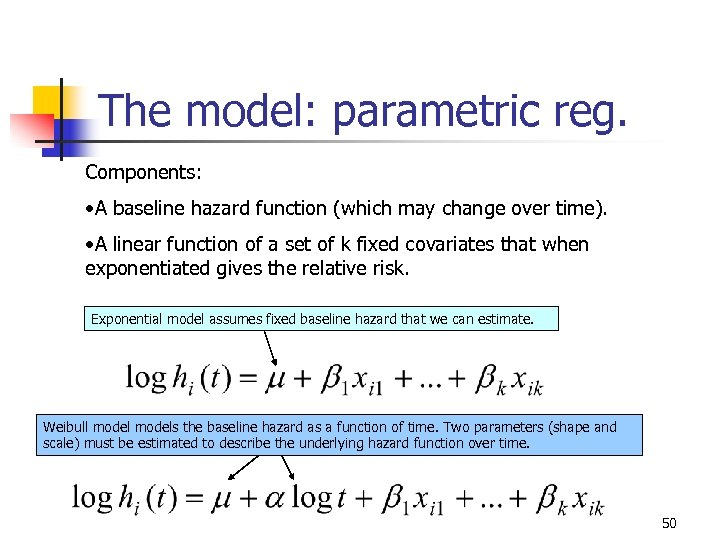The model: parametric reg. Components: • A baseline hazard function (which may change over time). • A linear function of a set of k fixed covariates that when exponentiated gives the relative risk. Exponential model assumes fixed baseline hazard that we can estimate. Weibull models the baseline hazard as a function of time. Two parameters (shape and scale) must be estimated to describe the underlying hazard function over time. 50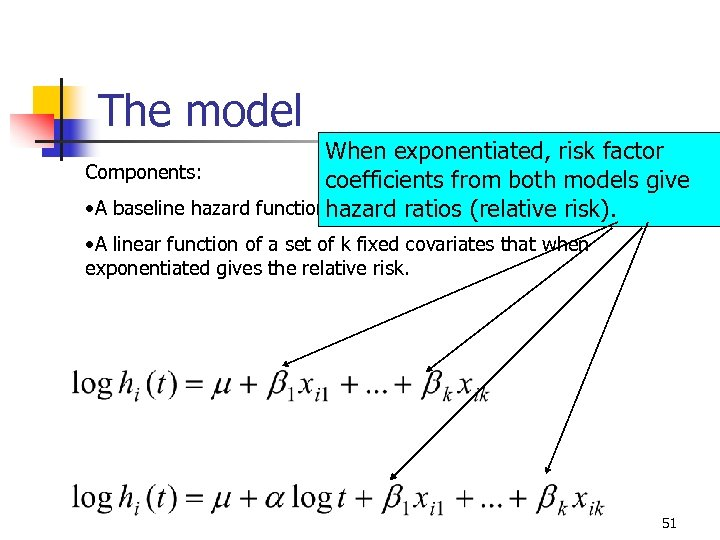The model When exponentiated, risk factor Components: coefficients from both models give • A baseline hazard function hazard ratios (relative risk). • A linear function of a set of k fixed covariates that when exponentiated gives the relative risk. 51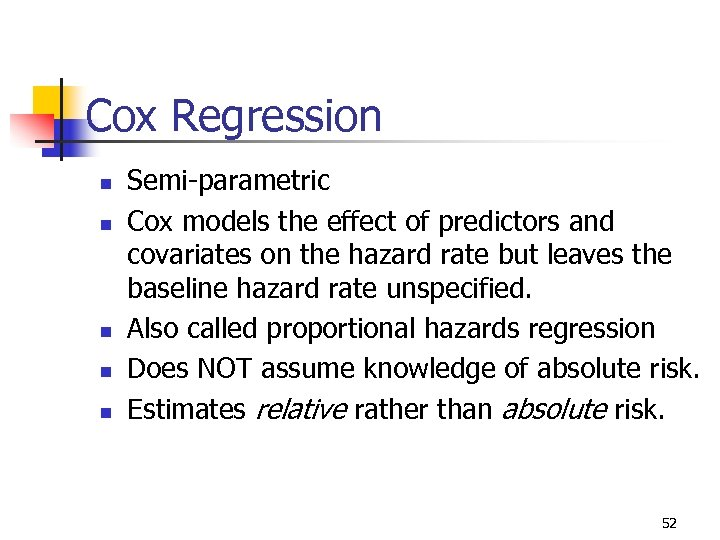Cox Regression n n Semi-parametric Cox models the effect of predictors and covariates on the hazard rate but leaves the baseline hazard rate unspecified. Also called proportional hazards regression Does NOT assume knowledge of absolute risk. Estimates relative rather than absolute risk. 52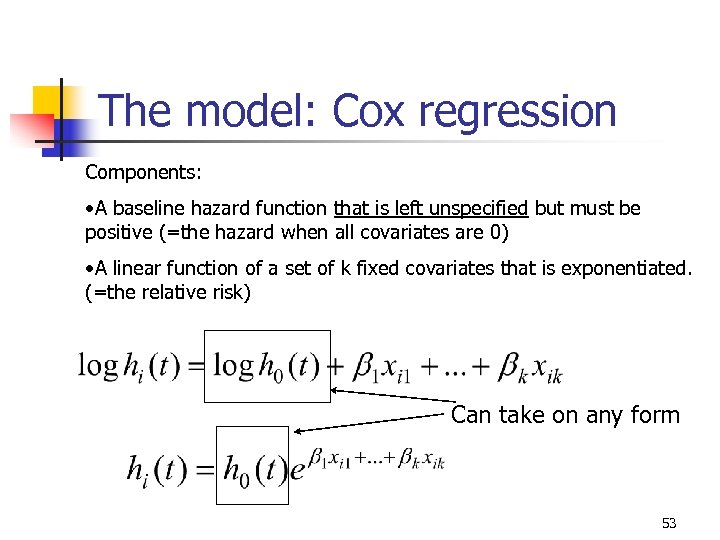The model: Cox regression Components: • A baseline hazard function that is left unspecified but must be positive (=the hazard when all covariates are 0) • A linear function of a set of k fixed covariates that is exponentiated. (=the relative risk) Can take on any form 53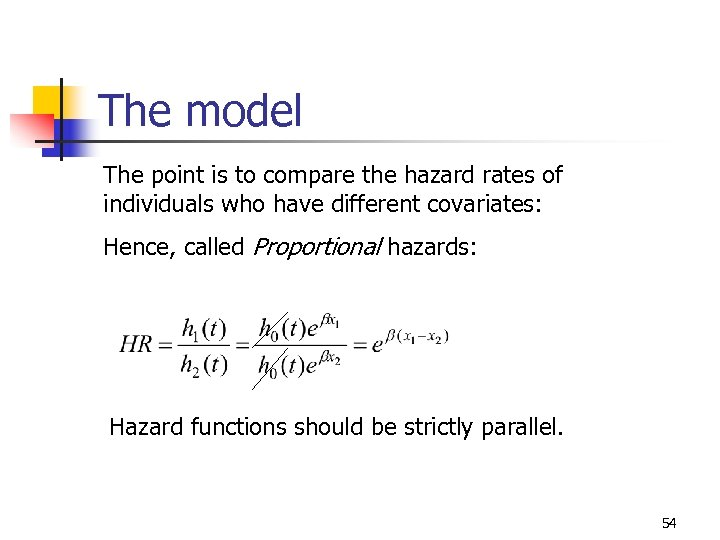The model The point is to compare the hazard rates of individuals who have different covariates: Hence, called Proportional hazards: Hazard functions should be strictly parallel. 54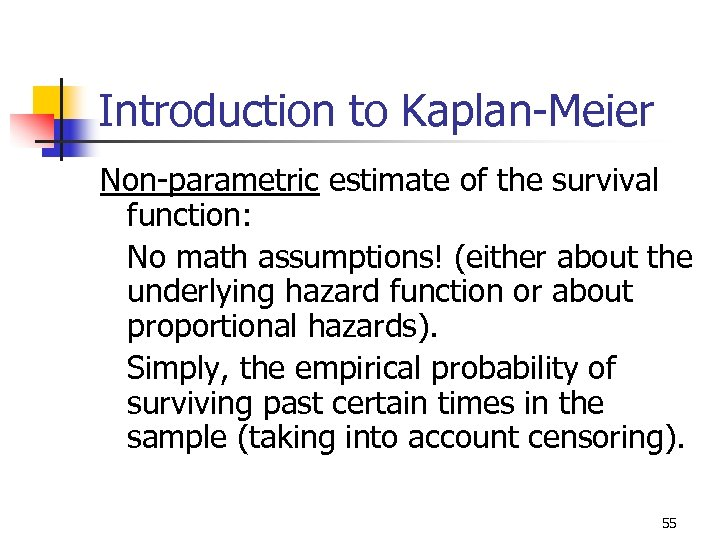Introduction to Kaplan-Meier Non-parametric estimate of the survival function: No math assumptions! (either about the underlying hazard function or about proportional hazards). Simply, the empirical probability of surviving past certain times in the sample (taking into account censoring). 55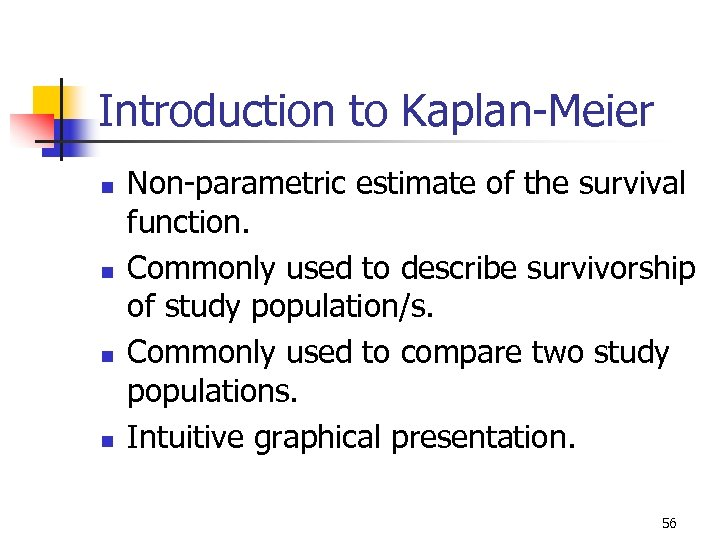Introduction to Kaplan-Meier n n Non-parametric estimate of the survival function. Commonly used to describe survivorship of study population/s. Commonly used to compare two study populations. Intuitive graphical presentation. 56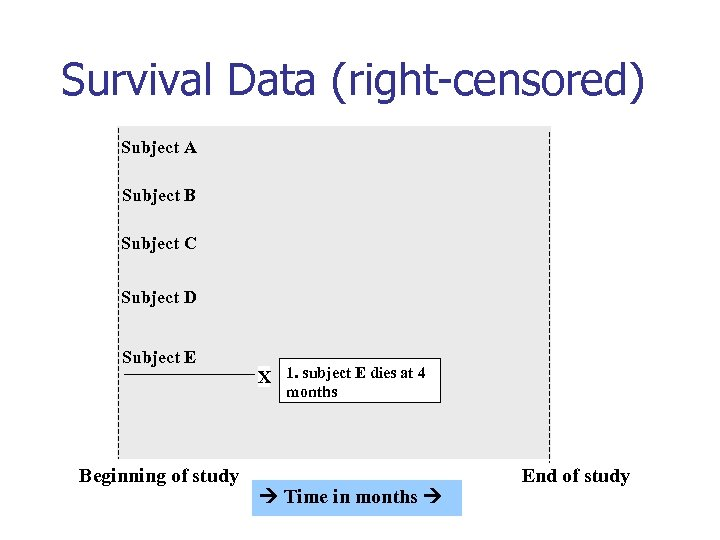Survival Data (right-censored) Subject A Subject B Subject C Subject D Subject E X 1. subject E dies at 4 months Beginning of study Time in months End of study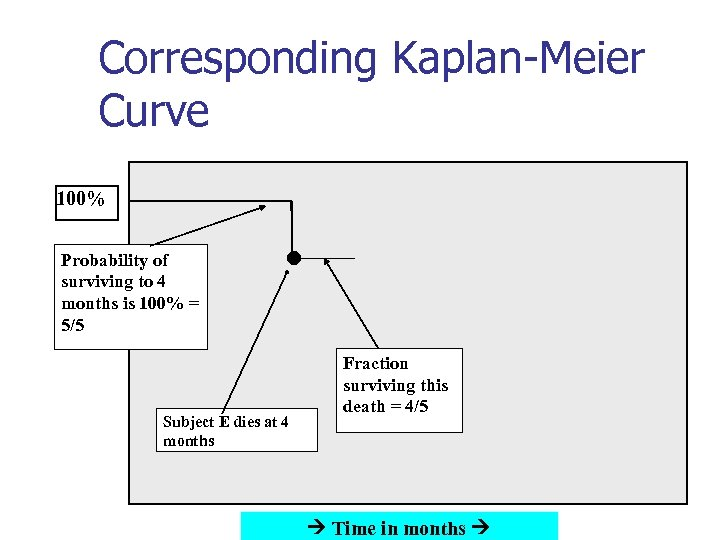Corresponding Kaplan-Meier Curve 100% Probability of surviving to 4 months is 100% = 5/5 Subject E dies at 4 months Fraction surviving this death = 4/5 Time in months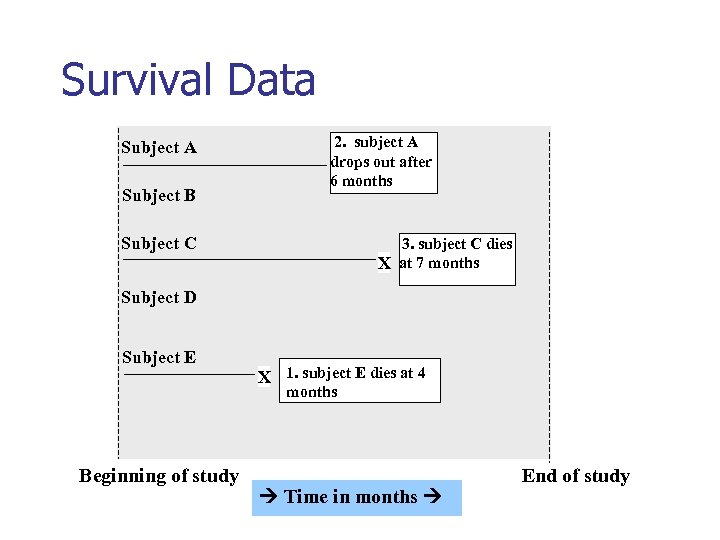Survival Data Subject A Subject B 2. subject A drops out after 6 months Subject C 3. subject C dies X at 7 months Subject D Subject E X 1. subject E dies at 4 months Beginning of study Time in months End of study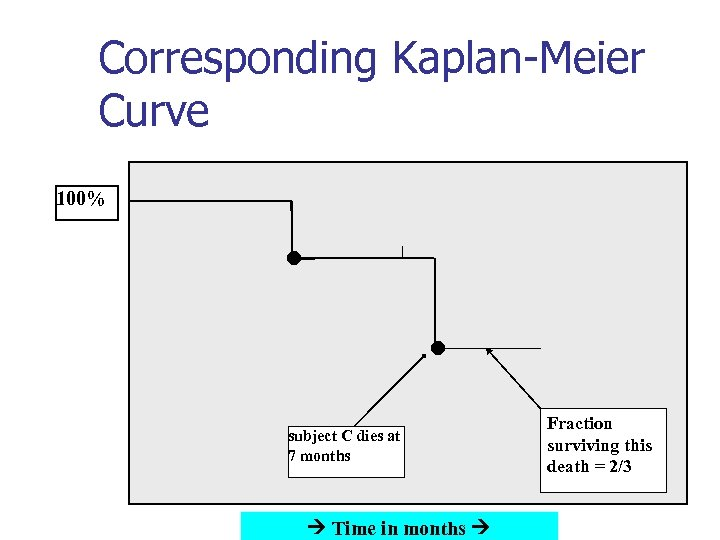Corresponding Kaplan-Meier Curve 100% subject C dies at 7 months Time in months Fraction surviving this death = 2/3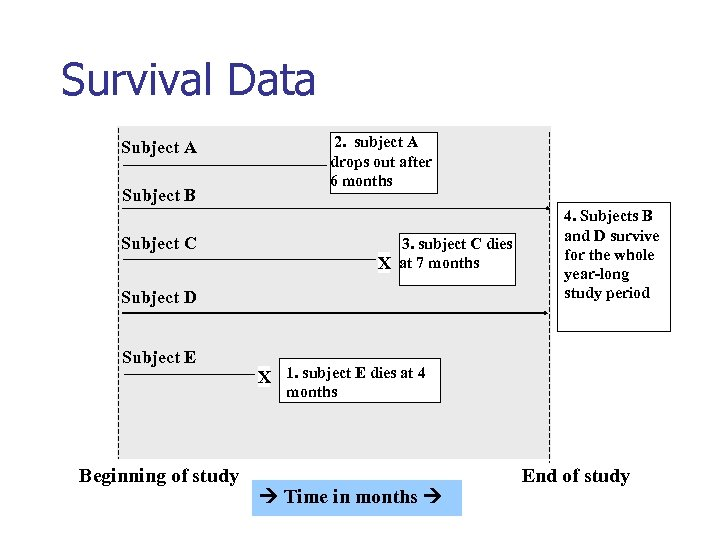Survival Data Subject A Subject B 2. subject A drops out after 6 months Subject C 3. subject C dies X at 7 months Subject D Subject E 4. Subjects B and D survive for the whole year long study period X 1. subject E dies at 4 months Beginning of study Time in months End of study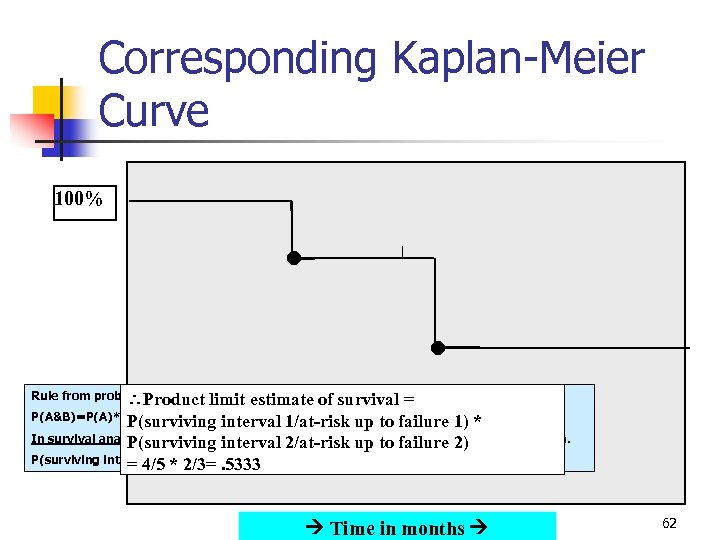Corresponding Kaplan-Meier Curve 100% Rule from probability theory: limit Product estimate of survival = P(A&B)=P(A)*P(B) if A and B independent 1/at risk up to failure 1) * P(surviving interval In survival analysis: intervals are defined by failures (2 intervals leading to failures here). P(surviving interval 2/at risk up to failure 2) P(surviving intervals 1 * 2/3=. 5333 = 4/5 and 2)=P(surviving interval 1)*P(surviving interval 2) Time in months 62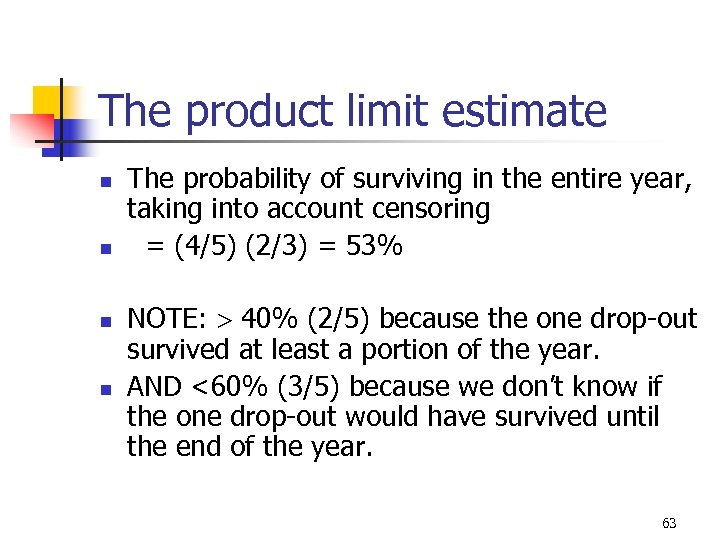The product limit estimate n n The probability of surviving in the entire year, taking into account censoring = (4/5) (2/3) = 53% NOTE: 40% (2/5) because the one drop-out survived at least a portion of the year. AND <60% (3/5) because we don’t know if the one drop-out would have survived until the end of the year. 63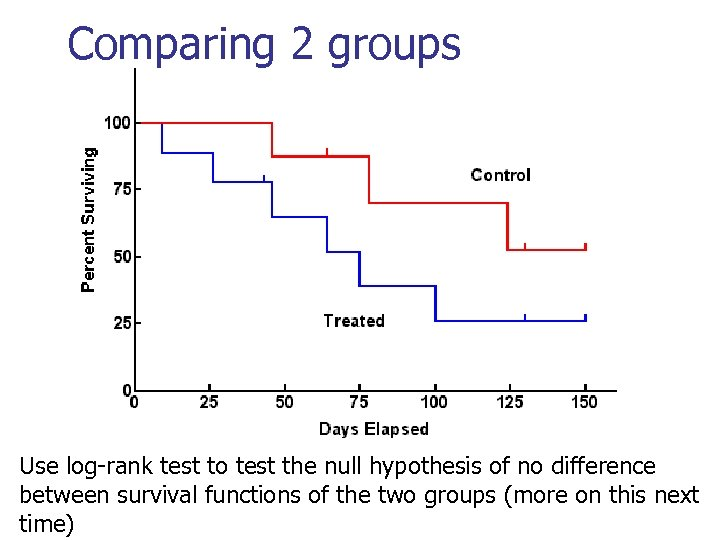Comparing 2 groups Use log-rank test to test the null hypothesis of no difference between survival functions of the two groups (more on this next time)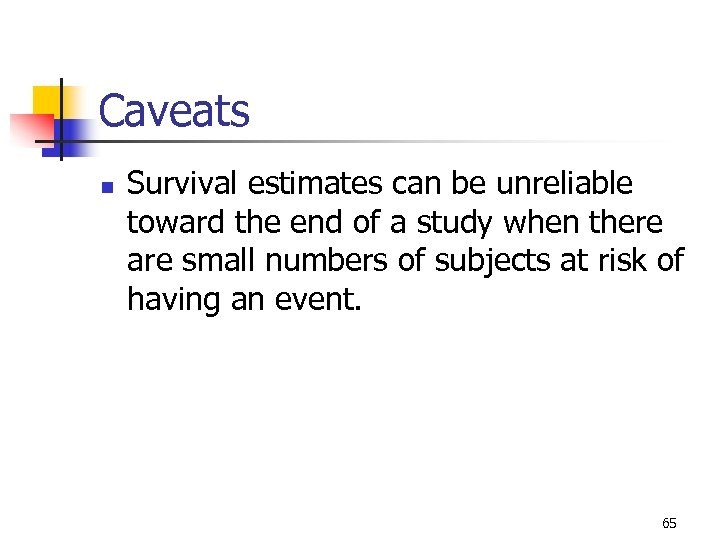Caveats n Survival estimates can be unreliable toward the end of a study when there are small numbers of subjects at risk of having an event. 65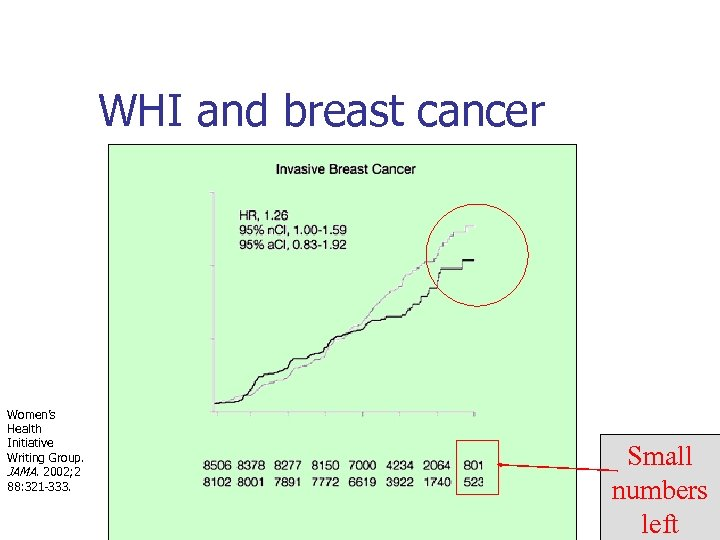WHI and breast cancer Women’s Health Initiative Writing Group. JAMA. 2002; 2 88: 321 -333. Small numbers left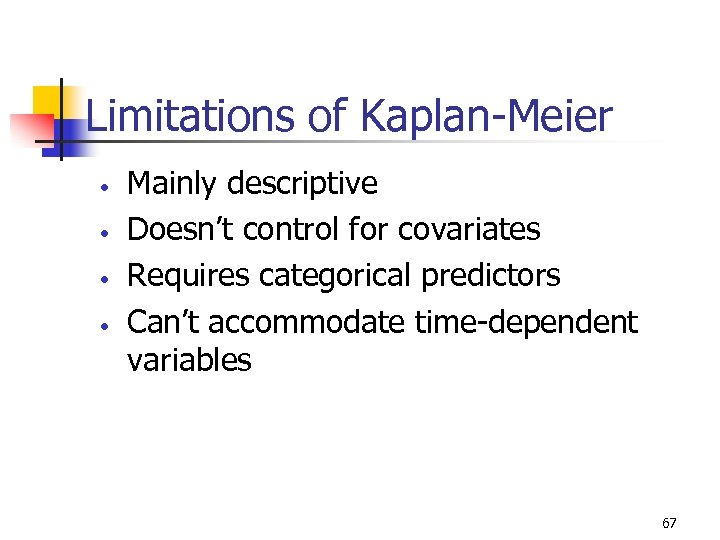Limitations of Kaplan-Meier • • Mainly descriptive Doesn’t control for covariates Requires categorical predictors Can’t accommodate time-dependent variables 67All about Kaplan-Meier next lecture… 68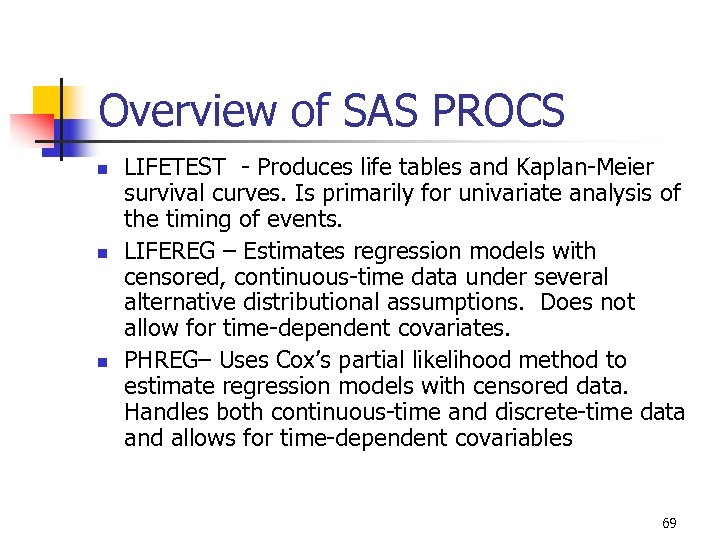Overview of SAS PROCS n n n LIFETEST - Produces life tables and Kaplan-Meier survival curves. Is primarily for univariate analysis of the timing of events. LIFEREG – Estimates regression models with censored, continuous-time data under several alternative distributional assumptions. Does not allow for time-dependent covariates. PHREG– Uses Cox’s partial likelihood method to estimate regression models with censored data. Handles both continuous-time and discrete-time data and allows for time-dependent covariables 69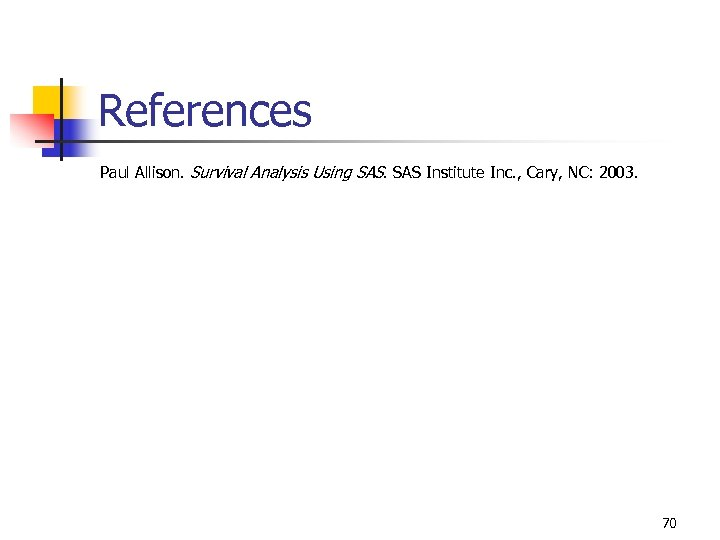References Paul Allison. Survival Analysis Using SAS Institute Inc. , Cary, NC: 2003. 70